Question

1. Chemical reaction rates are proportional to a rate constant, k, which changes with temperature according...

1. Chemical reaction rates are proportional to a rate constant, k, which changes with temperature according to the equation :

For a certain reaction:

Q = 8000 cal/mole R = 1987cal/mole K k0 = 1200 min-1

k = k0·e–Q/(R·T)

Find the values of k for temperatures from 100K to 500K in 50-degree increments. Create a table for your results. The table should contain two columns, with left column containing the temperatures and the right column containing the correcsponding values of k.

2. Generate 10000 Gaussian random numbers with mean of 80 and standard deviation of 23.5. Use the mean function to confirm that your array actually has a mean of 80. Use the std function to confirm that your standard deviation is

actually 23.5. Show the commands generating the numbers and the commands to compute the mean and standard deviation of the 10,000 numbers.

3. A small rocket is being designed to make wind shear measurements in the vicinity of thunderstorms. Before the testing begins, the designers are developing a simulation of the rocket’s trajectory. They have derived the following equation which they believe will predict the performance of the test rocket, where t is the elapsed time, in seconds:

height = 2.13·t2 – 0.0013·t4 + 0.000034·t4.751

Compute and print a table of time versus height, at 2-second intervals, up through 100 seconds. (The equation will actually predict negative heights. This, obviously will no longer be valid once the rocket hits the ground.For now, don’t worry about this physical impossiblity; we’ll justconcentrate on the math).

Use MATLAB to find the maximum height achieved by the rocket. Use an appropriately named variable to represent this value before you display it.

Use MATLAB to find the time the maximum height is achieved. Use an appropriately named variable to represent this value before you display it.

1. Code

Q = 8000;

R = 1987;

k0 = 1200;

n = 0;

for i = 100:50:500 % min:interval:max

n = n+1; %index purpose

K(n) = k0*exp(-Q/(R*i));

Temperature(n) = i;

end

T = table(Temperature',K') %display table

%Screenshot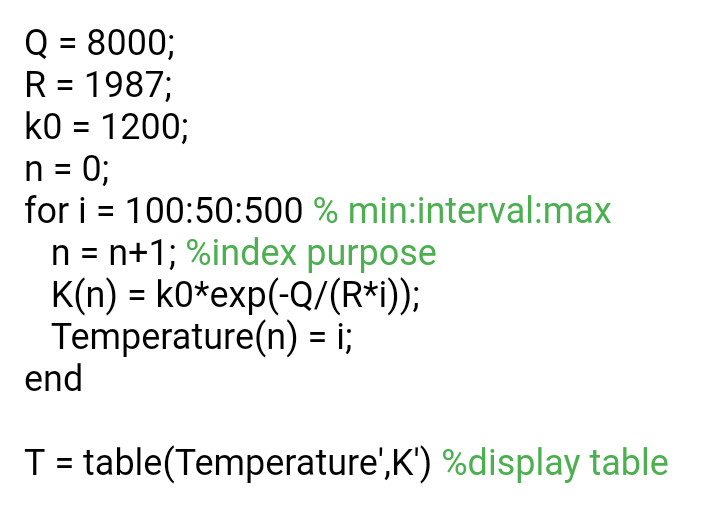.

2. CodeCode

Size = [1, 10000];

mu = 80; %mean

sigma = 23.5; %strandard deviation

r = normrnd(mu, sigma, Size); %Gaussian random numbers

disp('Mean of distribution is :')

M = mean(r)

disp('Error between expected mean and distribution mean is :')

M_error = abs(M - mu)

disp('Strandard deviation is :')

S = std(r)

disp('Error between expected std and distribution std is :')

S_error = abs(S - sigma)

%screenshot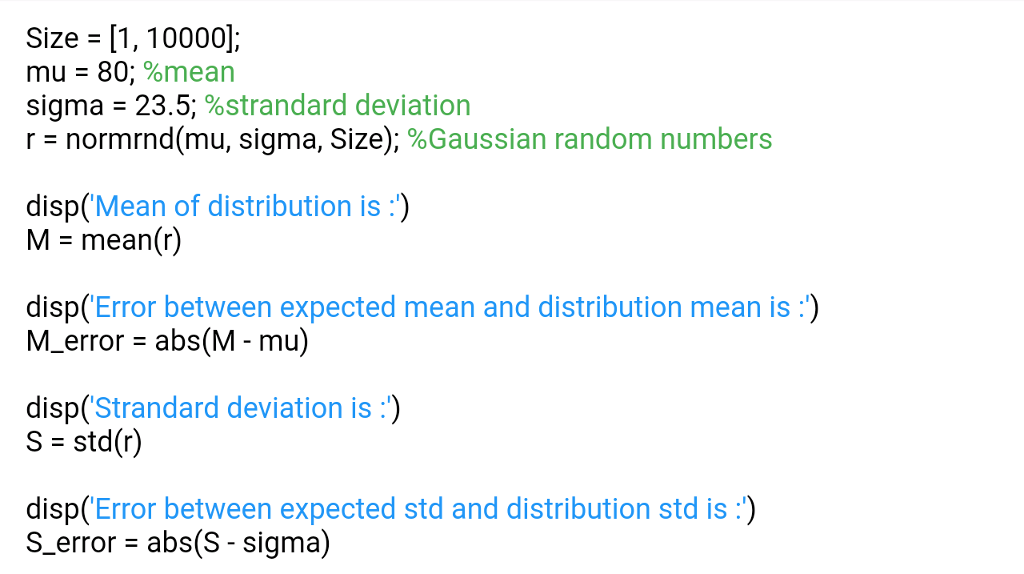.

3. Please pay attention to equation , Mentioned equation is not clear. So, I choose below equation, please enter right equation for right result.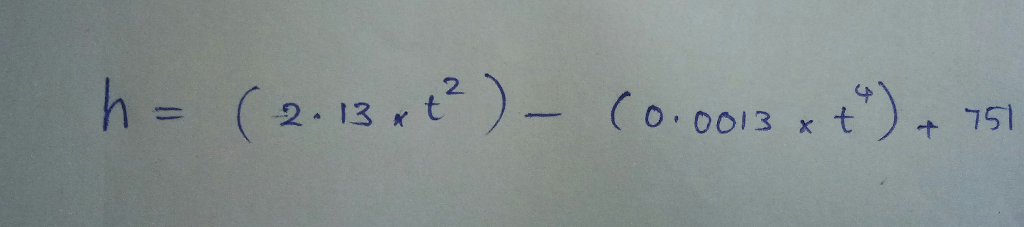Code:

n = 0;

for t = 0:2:100

n = n + 1;

Height(n) = (2.13*t^2) - (0.0013*t^4) + 751;

end

Time = 0:2:100;

disp('Maximum height achieved by Rocket is :')

max(Height)

T = table(Time, Height)

%Screenshot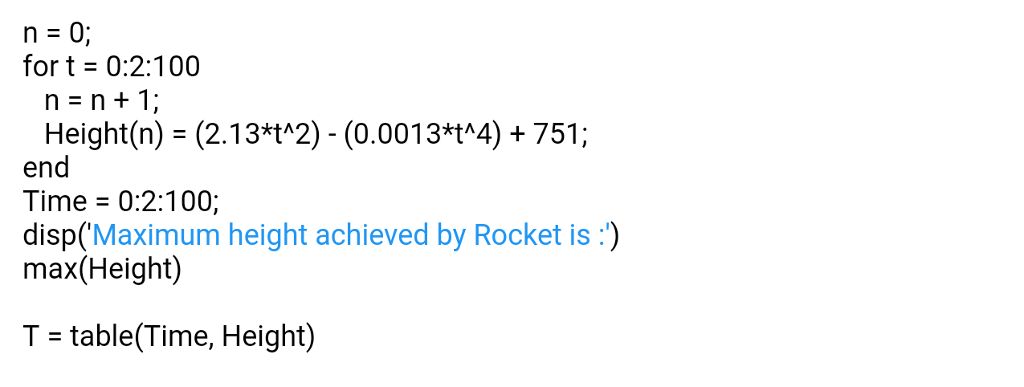Earn Coins

Coins can be redeemed for fabulous gifts.

Similar Homework Help Questions
• 1) What chemical is the titrant in this experiment? What chemical is the analyte in this experiment? Experi...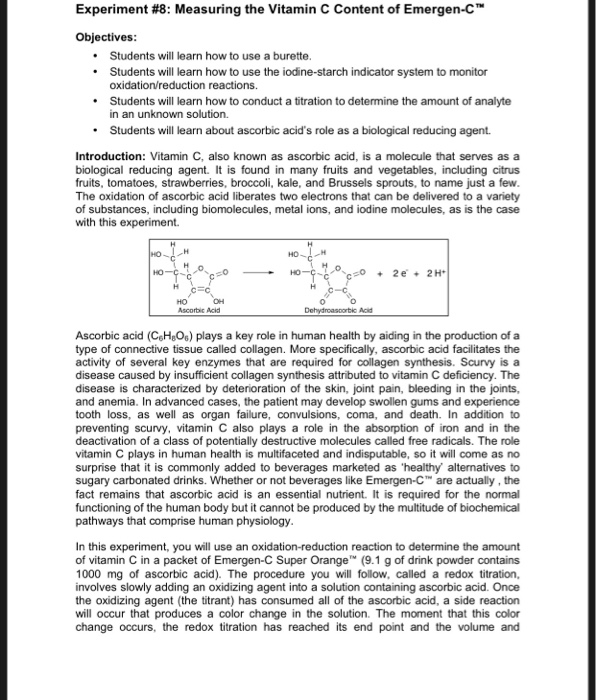1) What chemical is the titrant in this experiment? What chemical is the analyte in this experiment? Experiment #8: Measuring the Vitamin C Content of Emergen-C™ Objectives: • Students will learn how to use a burette. • Students will learn how to use the iodine starch indicator system to monitor oxidation/reduction reactions. Students will learn how to conduct a titration to determine the amount of analyte in an unknown solution. • Students will learn about ascorbic acid's role as a...